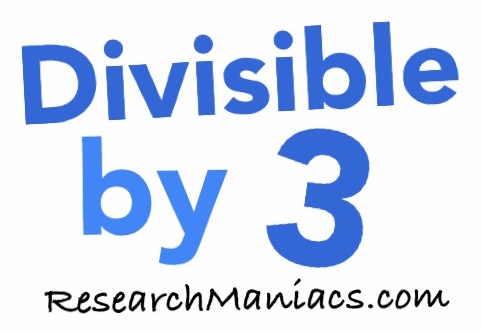Is 1765 divisible by 3?

 Is 1765 divisible by 3? In other words, if you divide 1765 by 3, will you get a whole number with no remainder? Of course, you could use a calculator to find out if 1765 is divisible by 3, but what fun would that be? To find out if 1765 is divisible by 3, we will add up the digits that make 1765 as follows: 1 + 7 + 6 + 5 = 19 We know that if the sum of the numbers that make up 1765 is divisible by 3, then 1765 is divisible by 3. Since the sum of the digits in 1765 is not divisible by 3, 1765 is also NOT divisible by 3. Thus, the answer to the question "Is 1765 divisible by 3?" is as follows: No Note: If you divide 1765 by 3, you get 588.33 which is not a whole number. Is 1766 divisible by 3? Can you do it on your own now? If not, go here for the next number on our list.Divisible by 3? Do you need to check another number? Enter a number below to see if it is divisible by 3.